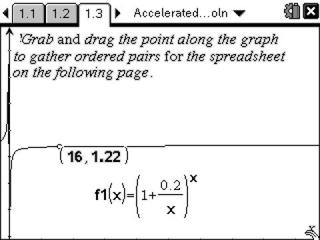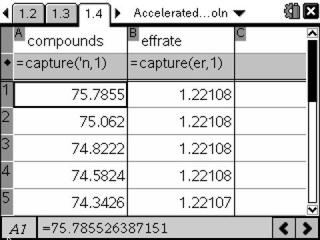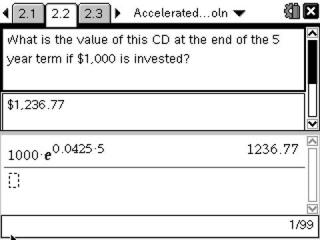Activities

••• Subject Area

• Math: Precalculus: Logarithmic Functions
• Math: Precalculus: Power Functions

• Author9-12

20 Minutes

• Device
• TI-Nspire™
• TI-Nspire™ CAS
• Software

TI-Nspire™
TI-Nspire™ CAS

2.0

• Report an Issue

Accelerated ReturnsActivity Overview

Students will compare periodic and continuous compounding and apply continuous compounding to a variety of problem situations.

Key Steps

•In this activity, students engage in an exploration of compounding interest. Students will apply continuous compounding of interest to a variety of problem situations.

Students grab and drag a point along the graph to populate a spreadsheet with the ordered pairs from the graph. Students explore both the graph and the spreadsheet to develop an understanding of what happens as the number of compounding periods gets infinitely large (as n approaches infinity).

•Students then compare the periodic compounding rate over various numbers of compounding periods per year to continuous compounding to find that as n gets infinitely large, the two formulas are equivalent.

•Students are presented with a variety of problems involving continuous compounding relating to personal finance. This is a great opportunity to help students better understand important financial concepts relating to investment and credit along with key math concepts.